## Introduction

The super-spreaders are the nodes in a network that can maximize their impacts on other nodes, as in the case of information spreading or virus propagation. The identification of these nodes is very important in many real-world domains, ranging from innovation diffusion1, viral marketing2, to epidemic disease identification and control3,4,5. Many methods, in particular centrality measure based methods, have been proposed in the past that aim to model and identify the most influential spreaders in complex networks. Among them, K-shell Decomposition method6,7,8 and Expected Force method9 have shown better performance than others under various epidemiological models. To evaluate the accuracy of the aforementioned measures various techniques has been employed. The most common one makes use of epidemic simulations10 from a single seed node, while the average infection size acts as the spreading characterization of the seed. Another possible way of evaluation is based on real-life tracking (e.g., in information spreading11) but, unfortunately, this approach is not always applicable due to the lack of data, for example, in transportation and computer networks. On the other hand, there are many networks where it is possible to quantitatively estimate the probability of spreading at the node level (e.g., based on such information as the number of passengers in a flight or number co-authorship papers) and bind it to the strength of connection between the nodes as a weight value associated with the edge10. This way allows for modeling and simulating the impacts of a spreader by taking into consideration the probability of node-level spreading. For instance, in the case of modeling information diffusion in a social network, the individual or meta-population level contact information (e.g., the duration or the frequency of the contact) can play an important role; this information will be reflected in the edge weights of the network12.

In essence, a centrality measure computes ranking score for the nodes of a network based on their connectivity characteristics. Various centrality measures have been used to predict the epidemic outcomes of the nodes, with the basic assumption that more centrally the nodes are located in the network, the greater spreading power they will have6,13,14,15. The well-known centrality measures, and the most frequently used in this field, include: Degree16, Strength17, Betweenness16,18, Closeness16, Eigenvector19, PageRank20 and K-shell6,7,21,22,23. A novel and more effective measure, known as Expected Force9, has been recently proposed with a particular aim for classifying nodes based on their influence in the network. This measure calculates the possible clusters generated from a fixed number of transmission events, and using entropy as aggregation of the clusters, it generates a node ranking. However, most of the previously proposed centrality measures take into account only the topological features of the network, without explicitly considering the varying strengths (or weights) of the connection strength, which are essential in modelling network diffusion processes. It has been shown that a natural extension to the weighted case is possible24 for all these measures (as detailed in Supplementary Information) except for K-shell where the ranking is computed from recursive pruning based on the node degrees. To address this issue, a weighted k-shell decomposition25,26 has been proposed, where the pruning is based on the neighbours’ connection strengths.

Another important aspect is concerned with determining the accuracy of the measures, that is to evaluate which measure can be considered as the best super-spreader predictor. In doing so, a susceptible-infected (SI) spreading simulation10 is typically run starting from each node in the network, so as to obtain a ground truth of the node spreading power. Thus, the problem is translated into that of evaluating which ranking, as calculated based on respective centrality measures, is better correlated with the one generated from simulations. A commonly used method is based on computing correlations (Pearson, Spearman and Kendall tau27). However, when the aim becomes the identification of the most influential node, just the top section of the ranking would be important. Besides, different methods, like imprecision function6 and recognition rate11, have also been proposed to evaluate a prefixed percentage of the ranking. This allows to have a better comparison because it increases the resolution on the ranking section where the super-spreaders are located. Generally speaking, for many networks, there is no single measure that over performs all the others among different percentages of ranking. For instance, different measures may perform better in different sections of the ranking15.

Due to the aforementioned observations, it remains a challenge to find which measure, if any, can better identify the super-spreader nodes. This is especially true if considering more realistic constrains, such as a spreading model based on the interaction strengths and an evaluation targeted to the top section of the ranking. To this extend, one can raise the following two questions: (1) Is it possible to find a measure that gives a consistent performance among different parts of the ranking for different kinds of networks? (2) Is it possible to find a corresponding patten between the best predictors and the characteristics of networks? To the best of our knowledge, there have been no studies in the literature that explicitly and adequately address these questions. In order to answer the aforementioned two questions, in this paper, we investigate a meta-centrality approach in which an aggregation method is used to combine different centrality measures and hence obtain a more robust performance in the super-spreader identification. Furthermore, we conduct a Laplacian spectrum analysis to characterize the networks and to evaluate their correlations with the results obtained from the aggregated measures. Throughout our evaluations, a set of real-word networks covering a broad range of domains has been used.

## Results

As mentioned above, there is no single centrality measure that consistently performs as the best predictor. This is because different measures have different objectives, and then based on them, they rank nodes in different ways. In an abstract sense, we may view the ranking computed from a certain centrality measure as that produced by an expert that evaluates certain features of a network. Thus, if we could aggregate the rankings (opinions) of different experts, we would be able to improve the final ranking results since each of the aggregated rankings brings its own contribution in the identification. A similar approach has also been adopted in other domains such as meta-search in Web engine28, biological databases29, and recommendation systems30. In doing so, it is essential to find the best aggregation of different rankings so as to obtain an improvement in the results. There have been some classical aggregation methods, such as Borda count method31,32, Kemeny-Young method33,34, and Median rank method. Some of the methods, for instance Kemeny-Young method, demand heavy computation even with a few (e.g., four) different rankings. In this regard, Borda count method requires relatively lighter computation, as it performs a scanning of all the rankings plus a sort. In addition, it also handles rankings with ties well35, which has been a major problem associated with some of the centrality measure rankings as they assign the same values to different nodes. The original Borda count method considers that every ranking has the same importance. A better performance can be achieved by using just a subset of the rankings or by using a weighted version of the Borda count32. Normally, this task involves training data to learn the optimal weighting schema. However, since it is not always possible to have training data, as in our case, other unsupervised models36 should be considered. In what follows, we first present a novel heuristic method, based on pairwise correlations, for selecting a subset of rankings so as to obtain an improved Borda count aggregation method. Next, we perform a series of experiments on real-network datasets to show the results of our proposed method. Finally, we correlate the topological features of the considered networks with the results as obtained from the improved Borda count aggregation by means of Laplacian spectrum analysis.

### The improved Borda count aggregation method

Generally speaking, Borda count method takes as input a set of ordered lists T = {τ1, …, τn}, where each list has the same C items in different orders. Let us denotethe position of the item z in the list τt. Then, the re-ranking value of an item z can be given as follows:where B is a vector with all the ranking items and |C| is the cardinality of the item set. Thus, the descending reorder of B represents the new aggregated ranking. In this basic form, each rank τtT is considered as being equally important, but there could be rankings that have a better accuracy than others. In our present case, the ordered lists correspond to the centrality measure based rankings, whereas the items C are the networks’ nodes. As mentioned above, the rankings generated by the centrality measures could be considered as opinions from different experts. Therefore, if a group of experts agree on the same subjects, their opinions could be considered more reliable. In reality, the opinions from experts who do not agree with the trend are still considered reliable, this is because they may still bring in useful alternatives. On the other hand, the situation in which experts are half agreed with each other will be considered unreliable, since their opinions are inconsistent with the mass. Thus, based on this idea of “social” aggregation, we formalize our heuristic method for selecting rankings.The method proceeds in the following steps: slicing, selection, and aggregation. Let M = [mij][0, 1]n×m be the correlation matrix, whereand ρ represents the Spearman correlation between the rankings τi and τj. It should be noted that this correlation also enables us to handle any possible disagreement among rankings by assigning it to a negative value. This may happen, for example, when we consider centrality measures such as clustering coefficient37,38. Indeed, this centrality assigns lower values to more central nodes, and hence it can lead to negative correlation values39. However, in the current work, we will not expect to encounter any large disagreement, since our rankings are based on the selection of those centrality measures that are known to be potential super-spreader identifiers, that is, they exhibit similar monotonic trends by their nature. This is also confirmed in all our experiments in that we have obtained only positive values of correlation except one case with a negative value very close to zero. Generally speaking, in handling such cases, we treat negative correlations similar to the uncorrelated ones (i.e., setting their values to zero).

The details of the three steps are as follows:

Slicing: This step attempts to select the subsets of ranking that are going to be used later in the aggregation step. To do so, we select a series of subsets for each row i in the matrix M. Let beand, where tb and ts are two positive constant thresholds. Thus, we define the following sets: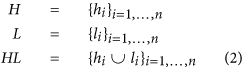where H represents the set containing high correlation subsets, L is the one containing low correlation subsets, and HL is the one having both. Note that different thresholds can create subsets with different cardinalities; we will further discuss about the threshold selection later. Naturally, one can expect that in this way, there could be many subsets, since each row could have different combinations of correlated rankings.

Selection: This step is designed to select the subsets of rankings that contain the most informative attributes. We discard some subsets of the ranking based on their entropy values as follows. Specifically, for each subset of the rankings, X = HLHL, we calculate its entropy based on the normalized correlation value. More formally, for each xiX, where i is the index of the selected subset row, we compute the following: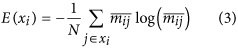where, and N = |xi| is a scaling factor. This division is used to tune the cardinality of the subsets, in order to pick solutions with a smaller number of rankings. Finally, after the calculation of the entropy, we select the subset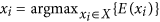and, to be more reliable, we also keep the subset with the second highest entropy value. Figure 1 presents a numerical example of this step.

Aggregation: With the above-mentioned two selected subsets, we calculate a new score for each node by performing the Borda count aggregation based on Equation (1). Thereafter, with the descending order of the list, we obtain the new ranking.

### Experiments on real-world networks

In order to evaluate the effectiveness of our proposed Borda count aggregation-based meta-centrality method, we conduct the super-spreader identification on 16 real-world networks from different domains. Table 1 shows the main features of the 16 networks. For each network, we first perform susceptible-infected simulations so as to establish a ground truth ranking for all the nodes in the network for the purpose of evaluation (See Methods). In doing so, we create a descending ranking I of nodes in which the first node has the largest infection influence. Next, we compute rankings based on centrality measures and use the results as the input of our aggregation method. The centrality measures used in the experiments consist of: Degree (CD), Strength(Cs), Closeness(CC), Eigenvector (CE), PageRank(CP), K-shell (CK), weighted K-shell (CKW), and Expected Force (CEX). It should be noted that here apart from K-shell and Degree centrality measures which are unweighted, all the others are calculated based on the edges’ weights (See Supplementary Information). We keep the two unweighted measures so as to bring in some contributions in the super-spreader identification from the topological perspectives. For consistency of notation, we let C* denote a centrality measure, S the node list sorted in a descending according to their ranking scores of C*, and A the rank generated from the aggregation.

To evaluate the accuracy performances of prediction by the considered centrality measures and by the aggregation, we compare only the top f nodes in the ranking generated from the ground-truth simulations and the one generated by a predictor. This evaluation method, also known as recognition rate11, can be expressed as follows: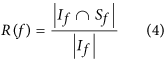where |*| represents the cardinality of a set, If the top section of the ranking generated from simulations, and Sf the top section from a centrality measure. In our experiments, we test six value of f, i.e., 0.05, 0.10, 0.15, 0.20, 0.25, and 0.50, and examine the mean and percentile of the recognition rates among them. This allows us to gain a better understanding of the accuracy performance, in particular, concerning the top section of the ranking. For each of the 16 networks, we evaluate all the considered centrality measures along with the two aggregated meta-centrality measures based on the aggregated rankings. Without loss of generality, the parameters of Equation (2) are set as: tb = 0.8 and ts = 0.3.

The above-mentioned two parameters allow for fine-tuning of our method. Here, we made heuristic choices in setting these values in order to select highly correlated as well as uncorrelated rankings. We conducted a series of experiments with values close to the selected ones, and as a result, we found that these values, as a general setting, could identify the correct centrality measures for the aggregation, consistently among all the networks. In other words, we would not make a customized selection of the parameters specifically for each network. Figure 2 shows the mean and percentile of the recognition rates among the considered f range. As shown in Fig. 2, the rankings generated from the aggregation results give the best predictions about super-spreaders for all the networks. In five networks (i.e., C. Elegans, Netsience, Astro-ph, Cond-mat, and Rail), there is a percentage rise of the mean value between 9%–18% as compared to the best single solution. In eight networks (i.e., FB, Geom, Hep-th, Metro, Coach, Advogato, AS, and Adolescent), the percentage rise is between 2–5%. And, in the remaining networks (i.e., US2013, US2015, and Names), the rise is around 1%. Furthermore, in almost all the aggregation results, we observe a decrease in the standard deviation. It should be noted that centrality measures are subject to large variations among different f. For instance, Fig. 3 shows the recognition rate values for all the considered centrality measures together with the best aggregation results in four networks. It should also be pointed out that some cases in our experiments, e.g., C. Elegans, Cond-mat, Hep-th, US2013, and US2015, as shown in Fig. 2, where the aggregation selected has the second highest entropy, denoted by A2 in the subplots, do not lead to the best mean but to a decreased standard deviation value. Therefore, we have used the mean value as the criterion of comparison, and hence the best aggregation from the early-mentioned selection step, denoted by A1 in the subplots, as the proposed solution. For the exact values of the percentages, the best single centrality measure among different f, and all the recognition rate plots, check Table S1 and Figures S1-4 in Supplementary Information.

### Network characterization based on Laplacian spectrum analysis

Our next task is to examine whether or not there exists a corresponding pattern (or correlation relationship) between centrality measures used for the aggregation and the networks of certain topologies. To achieve this task, we first characterize each of the networks using its Laplacian spectrum. The Laplacian spectrum analysis has been broadly used40,41 to describe the topology of the network, as it is capable of providing a model of the global topological features of a network, with no explicit reference to its individual nodes. Specifically, in this paper, we consider a Normalized Laplacian Matrix42, which is defined as, where A is the adjacency matrix of a network, D is the diagonal matrix of node weighted degrees, and I is the identity matrix. The eigenvalues of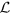are in the range of 0 ≤ λ1 ≤ … ≤λn ≤ 2, where n is the number of nodes in the network42. Thus, the Laplacian spectrum of a network refers to the eigenvalue distribution of its Normalized Laplacian Matrix. The Laplacian spectrum characterization provides the information about non-trivial characteristics of a network. There are three well-known characteristics40: small eigenvalues imply the presence of community structures43,44, large eigenvalues reflect the level of bipartiteness45, and a concentration of eigenvalues near to one indicates the presence of motifs41. At the same time, such a characterization allows for comparisons among networks with different sizes and network topologies. For a pair of networks, it is possible to define and compute a pseudo distance between their Laplacian spectra46 (See Methods) and hence to quantitatively determine their similarity. In our work, having defined a distance between two Laplacian spectra, we use a hierarchy clustering algorithm to group the networks into certain clusters depending on their similarities. This cluster analysis proceeds by considering each observation as a single cluster, and iteratively merging two clusters into a larger one based on a linkage criteria. In our current analysis, we have adopted a complete linkage criteria, which means using the couple with a maximum distance as the distance between two clusters.

Specifically, for each network, we calculate the eigenvalues of its Laplacian matrix. Then, by using the aforementioned methods, we identify four distinct classes of networks. Each identified cluster exhibits certain special network characteristics that influence the identification of super-spreaders. The first cluster that we have identified, i.e., the first sub-plot shown in Fig. 4a, may be referred to as the cluster of transportation networks, consisting of: Coach, Metro, and Rail networks. In this cluster, each of their histograms is almost symmetric, with a concentration of values in the two extremes. This means a high level of bipartiteness45. The aggregation in this network cluster uses CE and CC as its main components. This centrality couple are also present in other two clusters; indeed all of them have got some large eigenvalues. The second cluster that we have found, i.e., the second sub-plot in Fig. 4b, is composed of networks with the main part of their eigenvalues close to 1. These networks are: Advogato, AS, FB, US2013, and US2015 networks. The Laplacian spectrum of this cluster is typically developed from repeated additions and duplications of nodes and motifs41. In this case, two additional components are involved in the aggregation, namely, CKW and CEX. The networks in the third cluster, i.e., the third sub-plot in Fig. 4c, include Astro-ph, Cond-mat, Geom, Hep-th, Adolescent, and Netscience networks. Their eigenvalues tend to concentrate between 1 and 1.5. Similarly to the second cluster, the Laplacian spectra of these networks are also developed from recursive additions of triangle motifs47. The aggregation in this network cluster uses a new component CP. Finally, the forth cluster, shown as the fourth sub-plot in Fig. 4d, is made of two networks, C.Elegans and Names. Although they do not completely share the same spectrum, they are classified together because they do not have significantly large eigenvalues as in the cases of the other network clusters. In this cluster, the component CD is the common centrality.

In conclusion, we used the Laplacian spectrum to qualitatively characterize the aggregation results. As a result, for each of the four identified network clusters, we revealed a consistent pattern for the clustered network cases. That is, for each network that belongs to the same cluster, the same set of centrality measures in the aggregation could be found. It would be desirable to make an even stronger conclusion on the connection between network topologies and centralities (or an aggregation of them) that optimally identifies super-spreaders. However, due to the lack of sufficient network samples, it remains to be a future task to quantitatively establish and/or analyze such a connection.

## Discussion

Our work has shown that the aggregation of individual centrality measures results in an excellent predictor for super-spreaders, since it brings in contributions from each of individual ones so as to improve the identification accuracy. Most of the considered centrality measures work in a linear time, but not all. In fact, the closeness centrality does not work well for large networks. To overcome this problem, approximated version of this centrality has been proposed and can be used48.

The next important issue to highlight is about the simulation parameterization. Since our aim is to identify the most influential spreaders, the parameter α has been set equal to one, in order to perform a simulation that reflects the importance of the weights in the infection propagation. Indeed, if α is set to a large value, most of the nodes result in having similar outcomes, and thus we will not have a sufficient resolution to distinguish the importance of the nodes. Therefore, we have decided to fix the parameter α based on the following two considerations: First, we would like to bind the infection propagation only to the edge weights so as to reflect as much as possible the real importance of each node. Second, after a series of experiments, in each network, we have noticed that using different values of α would only increase the spreading power of every single node, while not affecting the final comparison of the nodes. Indeed, the model49 that we have implemented is made to bind the infection propagation to the edge weights (See Methods). Different methods have been proposed49,50,51 to achieve this task, but they were tailor made for particular applications and not suited to the problem of super-spreader identification.

Last but not the least, it should be pointed out that in our current work, we have chosen the SI model as the main spreading model. This is due to the consideration that it fits well with the task of super-spreader identification, in which infected nodes can spread freely until the entire network is fully covered. Nevertheless, it would also be desirable and interesting to examine the results of this work by adopting another commonly used model, i.e., the Susceptible-Infected-Recovered (SIR) model. When using the SIR model, the average numbers of Recovered nodes6 at the end of the epidemic spreading could be used for the ground truth ranking. In this study, in order to make sure that our method can also be applied to such a model, we have conducted several experiments by using our aggregation model on SIR simulations. The aggregated solution still gives the best results among all the networks. Interested readers are referred to more details in the Supplementary Information.

## Methods

In what follow, G(V, E) denotes an undirected, connected weighted network, where V represents the set of nodes, and E = V × V the set of edges, and wij represents the weight of the edge e(vi, vj). Let us denote with A and W the adjacency matrices of the network G, where Aij = 1 represents the edge e(vi, vj) and Wij = wij represents the weight of the connection.

As a measure of spreading power of a node, without loss of generality, here we describe a susceptible-infected (SI) simulation model. In the SI model, at the beginning, all the nodes are in the susceptible state (S) except the one that is in the infected state (I). At each time step of the simulation, the infected nodes will spread the infection to their neighbors, depending on the weights of the connecting edges. The simulation stops when all the network nodes are covered (labeled as I). Different models have been proposed to characterize an infection propagation process based on the connections’ strength49,50,51; in this paper, we adopt the most commonly used model49. In this model, the probability that a node i becomes infected at the time t is given by, where, α is a positive constant that describes the infection power and allows to tune the power and the speed of the infection, Ni(t) are the infected neighbors at the time t, and wM is the largest value of wij in the network.

To characterize the spreading power of each node, we run a batch of 100 simulations for each seed. In doing so, we record the infection ratio at each time step of a single simulation run in a set, until it has covered all the network nodes (note that the size of the set may vary among different simulation runs). Then, for each generated set, we take the average of its recorded values, and place it in a list Lsim (each value corresponds to a single simulation run). Finally, we calculate the average of the list Lsim previously generated, so as to obtain a single index that represents the spreading power of a node (more details can be found in Supplementary Information). Another way to characterize the spreading power of a node is to consider the average time of full coverage among different simulations. This method has been frequently used9, however with the above discussed method we can achieve a better characterization, without losing track of the average time of full coverage. A further discussion can be found in Supplementary Information under the SI evaluation section.

### Laplacian spectrum

The normalized Laplacian matrix of a weighted network G is defined as, where A is the adjacency matrix, D is the diagonal matrix of node weighted degrees, and I the is the identity matrix. Therefore, for each node u, vV, we have:where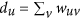. The eigenvalues ofdefine the spectrum of G, and they are in the range of 0 ≤ λ1 ≤ … ≤ λn ≤ 2, where n = |V|42. For each network Ni, we compute the corresponding spectrum of the Laplacian matrixand we define the corresponding eigenvalue histogram as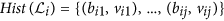, where bij represents the jth bin and vij its frequency. In our analysis, we fix the bin number to 200, such that every bin has a length of 0.01. Since every histogram has got the same number of bins, we can introduce a metric to formally quantify the distance between two spectra. Different metrics have been proposed to achieve this task, either by using a Gaussian kernel estimation52 or based on an Euclidean distance between the entire spectrum44. Instead, in this paper, we use the Earth mover’s distance (EMD), that is equal to Wasserstein distance, as the metric. This distance falls into the category of cross-bin measures as used to calculate distances between histograms. Computing EMD for multidimensional histograms generally requires the introduction of an optimization framework53. Since here we deal with a mono-dimensional histogram, the EMD becomes the distance between the cumulative histograms. More formally, the distance between two spectraandis defined as: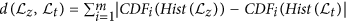, where CDFi is the cumulative histogram defined as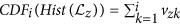. We choose this approach because it is simple and straightforward, and has been proven to be a pseudo metric as well as a graph spectrum distance46.

### Data Sets

In this work, all the networks are considered undirected, weighted, and connected. A brief description of the networks used is given as follows: (1) Names (nouns of the King James bible and their occurrences)54, (2) C. Elegans (weighted network representing a neural network)55, (3) Netscience (co-authorship network of scientists working on network theory and experiments)56 (4) FB (online community of students at University of California)57, (5) Advogato (online community for developers)54, (6) Adolescent (created from a survey that took place in 1994/1995, friendship choices by students)54, (7) Geom (authors collaboration network in computational geometry)58, (8) Astro-ph (co-authorships between scientists posting preprints on the Astrophysics E-Print Archive)59, (9) Hep-th (co-authorships between scientists posting preprints on the High-Energy Theory E-Print Archive)59, (10) Cond-mat (co-authorships between scientists posting preprints on the Condensed Matter E-Print Archive)59, (11) and (12) US air passengers 2013 and 201560, (13) AS (snapshot of Autonomous System network, where the weights representing the visit-counts in the period of time)61, and (14), (15) and (16) Metro, Coach and Rail respectively (network map of UK transportation system, where the edge weights are the average minutes of travel)62,63.